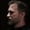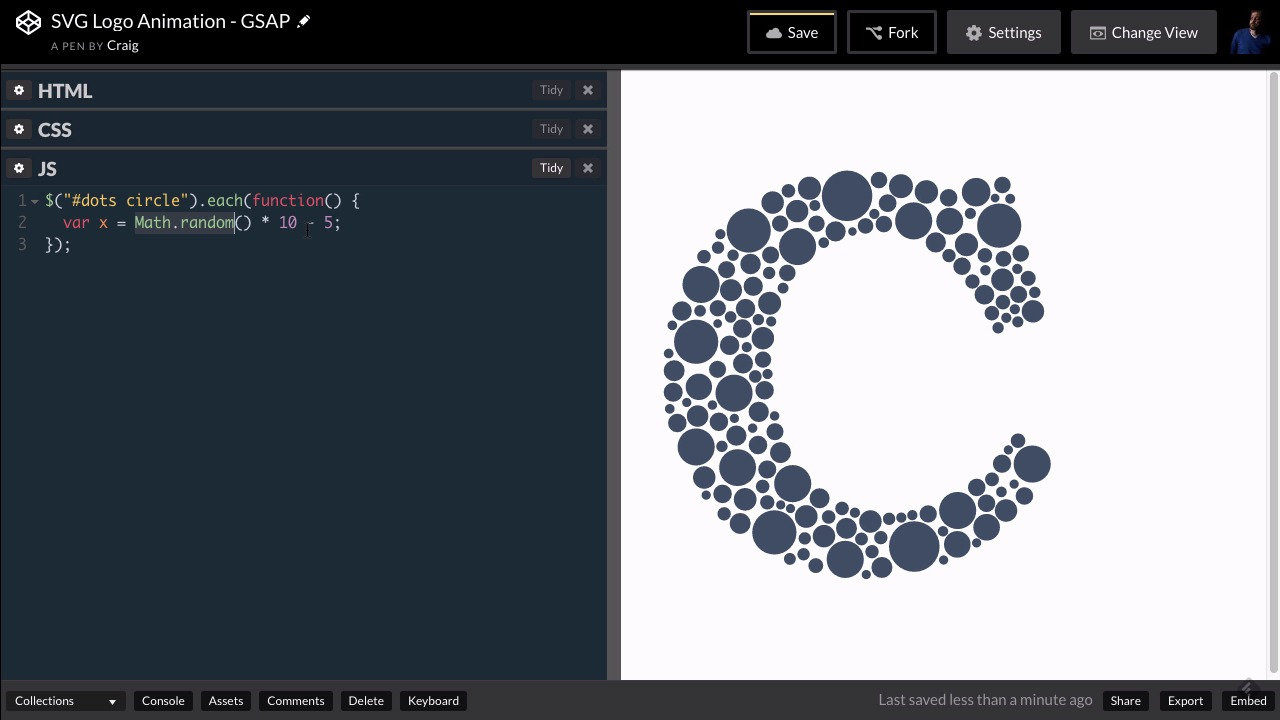Lessons: 25Length: 3.1 hours• Overview
• Transcript

# 3.3 Randomizing Position and Opacity

It’s time to learn how to randomize the positions of dozens of objects in order to start your SVG animation.

## 5.Conclusion1 lesson, 00:46

### 3.3 Randomizing Position and Opacity

Hello and welcome back. In our last lesson, we set up the styles for our SVG file and we can't see anything right now because remember, we turned off the opacity for those items for the text as well as the circles that make up the larger part of our logo. And now that we've done that, I want to randomise the position of the dots within our logo. So for now, I'm gonna turn this opacity back on and return it back to one. Before you do that, go ahead and make sure that you fork your copy of the code pen so that now we have a new fresh copy to work with and then let's go back to our CSS. I'm gonna turn on the opacity, I'm going to turn that back up to one, so that we can see all of the circles in the larger part of our logo. So then let's jump into JavaScript. Now I want to first of all randomize the position of each of the dots in our logo, each of these circles. And so since we're doing this for each one of these circles individually, we can do that in jQuery by pointing to our dots. And again, if we go into our HTML, those are all contained within this group, it has an ID of dots in our SVG. We have a bunch of circle elements and those circle elements are what we want to target now. So we're gonna point to the dots ID and inside that, we're gonna point to the circle element. So let's jump into JavaScript, and inside our jQuery selector, we're gonna put a set of quotation marks and I'm gonna point to the dots ID by typing pound dots. And then we'll point to the circle elements inside that by typing space circle. And then we want to run a function for each one of these circles. So we're going to use the jQuery each method. So inside the parenthesis for the each method, we're gonna create a new function. So we'll type the word function, open and close parentheses and then an opening and closing curly bracket and we'll nudge that closing curly bracket down a couple of lines and don't forget to put your semicolon at the end of that statement. So in between the curly brackets for that function, we're going to first of all randomise the opacity of each of these items. So in between the curly brackets for this function, I want to randomize the position of each of these items. And we're gonna randomize the x-coordinate as well as of the y-coordinate, we're gonna do those separately. So let's start by just storing the value, the random value that we're gonna come up with. Let's store those in a variable. So we'll call the first variable x for our horizontal positioning and I'm gonna set this. First of all, let's take a look at the Math.random function. The Math.random function returns a value between 0 and 1. If we want a number between 0 and 10 for example, we would just say Math.random times 10 and that would give us a random number between 0 and 10. Here I want a random number between negative 250 and positive 250. So that gets a little bit trickier because we're not starting with zero. If we want a random number between 0 and 10, again, we would just multiply it by 10. But what if we wanted between negative five and positive five? So we still have a range of 10, but then we would just subtract 5 from it. So the way this works is this would be your range. So this is a range of ten, again from negative five to positive five and this would be your lower limit of that range. So negative five would be the lowest possible value. If Math.random returned 0, 0 times 10 is 0 minus 5 is negative 5, and the highest possible value would be a positive 5. So we wanna do the same thing here except we want anything from negative 250 to positive 250. So that's a range of 500, so we're gonna multiply this by 500. So Math.random times 500 will give us a random number between 0 and 500 and then if we just subtract 250 from that and that gives us a random number between negative 250 and positive 250. And I'm gonna do the same thing for y. So I'm gonna copy that and then on the next line, we'll just paste it and change x to y. So now we have two separate variables with two different random numbers in them, and then we can use TweenMax to set the position of those items of those circles in the xy coordinates that we just randomized. So the way we would do that is we would point to TweenMax with a capital T in Tween and a capital M in Max dot and again, we're not animating now, we're just setting the values. So we're gonna do TweenMax.set and we're going to set the xy coordinate for whatever circle we're currently looping through inside this each function. So we're gonna set this comma space and then inside curly brackets, we're gonna set our x and y values. So x, we're gonna set equal to the x variable that we just created up here. If you want to make that a little less confusing, we could call this something different like xPOS or xPosition. That way, we don't have two x's right next to each other. That makes it a little more clear hopefully to those of you who might have been confused by that. So let's change x to xPosition and then y to yPosition. So we have x, we're gonna set that equal to our xPosition variable and then y we're gonna set equal to our yPosition variable. And now our dots have been completely randomized. And what we wanna do is we want to animate these two values back to zero. And you'll notice that when we set them to zero, all of the circles end up back in their original starting place. So I'm gonna hit undo a couple of times to get back to our random numbers, and every time you refresh this, they're gonna be in a different location. So this animation will be different every time you watch it, because these circles will be randomized in different locations. The next thing I'm going to do is I'm gonna randomize the opacity. So I'm gonna create another variable up here called opacity, and we're gonna set this to a value of Math.random and I want this to be a value between 0.4 and 1, any where between 0.4 and 1. So that gives us a range of 0.6. If you do 1 minus 0.4, that gives you 0.6. So we're gonna multiply Math.random by 0.6 and then we'll add the minimum value which is 0.4, and and close that off with a semi-colon. So we've stored that in a variable called opacity. And so now remember, these are all gonna be invisible when we first start this animation, but as we animate them on, we're gonna animate them to whatever value we see here. So it's gonna go from 0 to whatever random value shows up here. And if we just wanna test and see what that looks like, we can go inside this TweenMax.set and set our opacity to the value that we stored in that variable that we called Opacity. And now they will be spread out, and the opacity is also randomized. So again, this isn't where we're gonna stick this particular value, we're gonna do this inside the animation itself. But I just wanted to show you what that's gonna end up looking like, so let's get rid of that right there. Let's leave the x and y positions randomised and in the next lesson, we'll start animating this. Thank you for watching and I'll see you then.

Back to the top